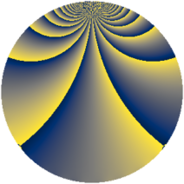# Properties

 Label 1694.2.vLevel $1694$ Weight $2$ Character orbit 1694.v Rep. character $\chi_{1694}(15,\cdot)$ Character field $\Q(\zeta_{55})$ Dimension $2640$ Sturm bound $528$

# Related objects

## Defining parameters

 Level: $$N$$ $$=$$ $$1694 = 2 \cdot 7 \cdot 11^{2}$$ Weight: $$k$$ $$=$$ $$2$$ Character orbit: $$[\chi]$$ $$=$$ 1694.v (of order $$55$$ and degree $$40$$) Character conductor: $$\operatorname{cond}(\chi)$$ $$=$$ $$121$$ Character field: $$\Q(\zeta_{55})$$ Sturm bound: $$528$$

## Dimensions

The following table gives the dimensions of various subspaces of $$M_{2}(1694, [\chi])$$.

Total New Old
Modular forms 10720 2640 8080
Cusp forms 10400 2640 7760
Eisenstein series 320 0 320

## Trace form

 $$2640q - 2q^{2} - 4q^{3} + 66q^{4} + 6q^{6} - 2q^{8} - 652q^{9} + O(q^{10})$$ $$2640q - 2q^{2} - 4q^{3} + 66q^{4} + 6q^{6} - 2q^{8} - 652q^{9} + 10q^{11} + 18q^{12} + 128q^{13} + 40q^{15} + 66q^{16} - 12q^{17} + 2q^{19} + 8q^{21} + 34q^{22} - 8q^{23} + 6q^{24} + 102q^{25} - 10q^{27} + 16q^{29} + 20q^{30} + 88q^{31} + 8q^{32} - 34q^{33} - 28q^{34} - 4q^{35} + 64q^{36} + 16q^{37} + 164q^{38} + 24q^{39} - 12q^{41} + 52q^{43} - 24q^{45} + 8q^{46} - 28q^{47} - 4q^{48} + 66q^{49} + 10q^{50} + 156q^{51} + 16q^{52} + 28q^{53} - 64q^{54} + 148q^{55} + 32q^{57} + 72q^{58} + 70q^{59} + 16q^{60} - 24q^{61} + 24q^{62} + 66q^{64} + 124q^{65} + 14q^{66} + 48q^{67} - 12q^{68} + 48q^{69} + 36q^{70} + 88q^{71} - 10q^{72} + 60q^{73} - 52q^{74} - 30q^{75} + 38q^{76} - 8q^{77} - 64q^{78} + 36q^{79} - 690q^{81} + 74q^{82} - 30q^{83} + 8q^{84} + 168q^{85} + 26q^{86} - 72q^{87} + 56q^{88} - 60q^{89} + 204q^{90} + 168q^{91} - 8q^{92} + 60q^{93} + 144q^{94} + 208q^{95} - 4q^{96} + 46q^{97} + 8q^{98} - 30q^{99} + O(q^{100})$$

## Decomposition of $$S_{2}^{\mathrm{new}}(1694, [\chi])$$ into newform subspaces

The newforms in this space have not yet been added to the LMFDB.

## Decomposition of $$S_{2}^{\mathrm{old}}(1694, [\chi])$$ into lower level spaces

$$S_{2}^{\mathrm{old}}(1694, [\chi]) \cong$$ $$S_{2}^{\mathrm{new}}(121, [\chi])$$$$^{\oplus 4}$$$$\oplus$$$$S_{2}^{\mathrm{new}}(242, [\chi])$$$$^{\oplus 2}$$$$\oplus$$$$S_{2}^{\mathrm{new}}(847, [\chi])$$$$^{\oplus 2}$$